• 0

# Bayes' theorem

Go to solution Solved by Sharad Talvalkar,

Bayes' theorem

Bayes' Theorem describes how the conditional probability of each of a set of possible causes for a given observed outcome can be computed from knowledge of the probability of each cause and the conditional probability of the outcome of each cause

An application-oriented question on the topic along with responses can be seen below. The best answer was provided by Sharad Talvalkar on 05th August 2019.

Applause for the respondents- Mohamed Asif, Manjula Pujar, Sharad Talvalkar, Ram Rajagopalan & Sreyash Sangam

## Question

Q﻿﻿. 182  Explain Prior Probability and Posterior Probability along with application of Bayes' theorem in a business scenario.

Note for website visitors - Two questions are asked every week on this platform. One on Tuesday and the other on Friday.

## Recommended Posts

• 0
• Solution

Explain prior probability & posterior Probability along with application of   Bayes Theorem in a business scenario.

Prior probability : Probability is an intricate subject. Therefore, initially the concepts of Probability  are introduced / explained  with the  help of orderly examples where the outcomes are known to us by applying simple logic/ common sense. E.g. Tossing a fair coin, rolling an unbiased dice, drawing a card from a deck. In  all these aforesaid cases we can make our probability statements even prior to conducting any experiment.  We know that the probability of getting a Head when a coin is tossed is 0.5 ( 50%) Hence such classical cases are known as Prior Probabilities where the outcome is known even before the experiment is conducted.

Classical approach defines the probability of getting either Head or Tail when a coin is tossed as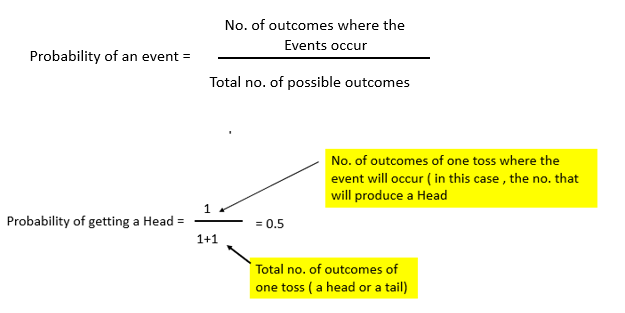This approach to probability is useful when we deal with Coin tosses, card games , dice game etc. Real life situations in management are not  so straight forward & therefore one has to define probability in a different way.

Posterior Probability :     At the beginning of the World Cup Cricket Match 2019, Indian Fans were very confident (99%)  that India will win the world cup. As the matches progressed, some key players like Shikhar Dhawan , Vijay Shankar got  injured & they could not participate .  Hence after getting this additional information, Indian Fans revised the probability  ( let’s say 80% )of winning a world cup. This revised probability after getting additional information is known as Posterior Probability.

A similar situation also occurs in a business scenario. A shopkeeper may order various colors of Jacket ( Blue, Black, Grey etc.) based on the past consumption pattern. As time progresses, he may notice that the sale of Jackets is not as per his expectation & so after getting this input the shopkeeper  may change his ordering pattern of Jackets. This revised ordering pattern is an example of Posterior Probability.

Bayes Theorem: Bayes formula for conditional probability under dependence is as follows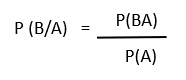Let us now understand the application of Bayes Theorem in a business scenario with the help of following example

Suppose there are three machines ( M1,M2 & M3), each of them producing a same component, say X. Production from M1, M2 & M3 is 40%, 49% & 11%. If there is a customer complaint from the market what is the probability that it is from M1, M2 & M3.

Using simple logic, we can say that the probability of defective coming from M1,M2 & M3 is 0.4, 0.49 & 0.11 respectively.

Now we have additional information that the defectives from M1,M2 & M3 are 0.5%, 3% & 2%. In this scenario when a complaint  comes from the market what is the probability that the defective is coming from M1,M2 & M3 .

Using Bayes Theorem, we can say that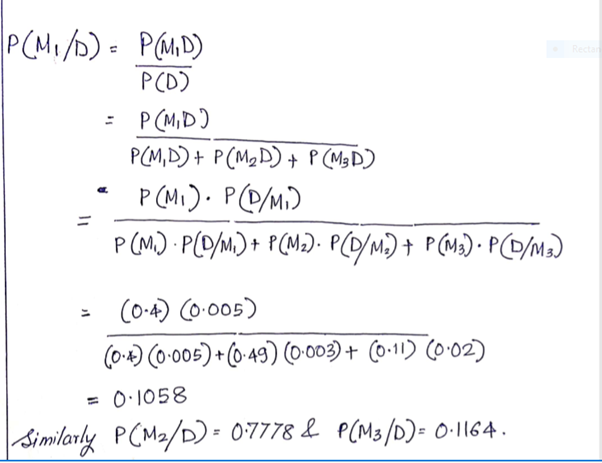Using Bayes Theorem, we can say that

In the above example our initial probability of getting defectives from M1,M2 & M3 was 0.4, 0.49 & 0.11.respectively. This probability is the Prior Probability.

Later, after getting additional information that the defectives from M1,M2 & M3 are 0.5%, 3% & 2% we have revised the probability of getting defectives from M1,M2, M3 to 0.1058, 0.7778 & 0.1164. This revised probability is known as Posterior Probability.

--------------------------------------------------------------------------------------------------------------------------------------------------------------------------------------------------------------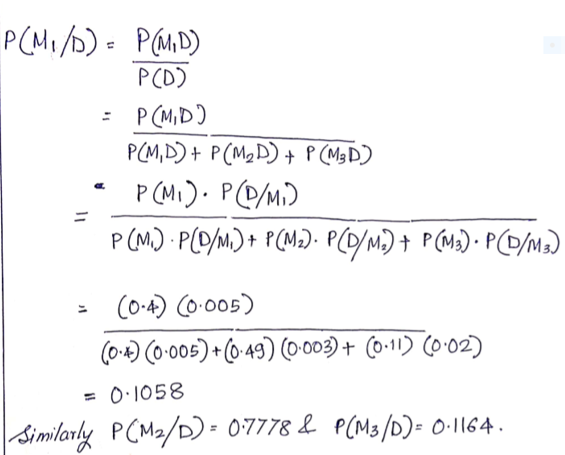##### Share on other sites
• 0

Covering some basics:

Posterior Probability (Conditional Probability):

We use this, when we have new considerations based on recent updated data and wanted to update the probability of the event.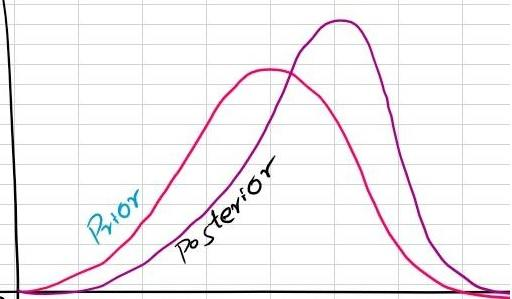Under Normal Distributed prior and likelihood probabilities, we shall be able to describe posterior probability with a function. This is referred as Closed Form Solution.

It is often referred as revised probability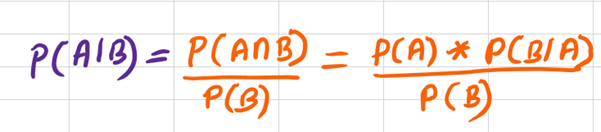Where,
A and B are events;
P(A) is Probability of A occurring;
P(B) is Probability of B occurring;
P(A|B) is Conditional probability of A (given that B occurs)
P(B|A) is Conditional probability of B (given that A occurs)

P(A) & P(B) are Prior Probabilities

Note:
Posterior probability is calculated by updating prior probability

To make it simple, Posterior Probability = Prior Probability + New Evidence

Considering an example of Gold Rate and Rupee Strength,
Suppose, gold rate increased 70% of the time and gold rate decreased 30% of the time.
i.e., P(Increase) = 0.7; P(decrease) = 0.3

In recent past after demonetization, given rupee lose strength against dollar, gold price increased 80% of the time and given rupee gained strength, gold price decreased 40% of the time
i.e., P(loss | Increase) = 0.8 and P(Gain | decrease) = 0.4

Here, P(Increase) and P(decrease) are Prior Probabilities
And, P(loss | Increase) and P(Gain | decrease) are Conditional Probability

Now getting into results,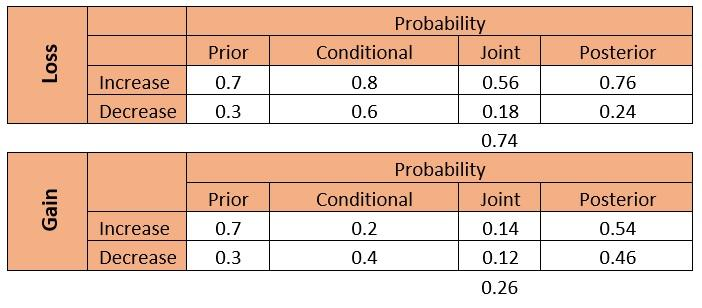So, Probability of Gold price will increase given for weak rupee is 0.76

We can apply Bayesian approach in below business scenarios widely to predict outcomes,

• Marketing and R&D
• Pricing Decision
• New Product Development
• Logistics
• Promotional Campaigns

Taking Bayesian stat to next level, is to leap into MCMC, Markov Chain Monte Carlo methods.

This methods helps in finding posterior distribution of the metrics. These algorithms generates simulations to find the metric parameter.

We can write simple codes to get the system calculate the probability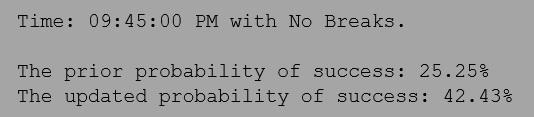(Python code)

##### Share on other sites
• 0

Bayes Theorem

Bayes theorem is about probability of 2 or more parameters.

If parameter of distribution, can be estimated which can not be fixed one. It may be random variable.

Bayes theorem works on probability rules

Normal probabability :

 B

 A

If A and B are two boxes and we have to select one box

Then probability of A and B

Probability(A)è P(A) = 1/2 è A/(A+B)

Probability(B)è P(B) = 1/2 è B/(A+B)

Suppose  there are 3 Boxes A Band C

Then P(A)=1/3     P(B)=1/3  P(C)=1/3

Let’s say possibilities of selecting box A is 55% , box B is 30% and box C is 15%

Then

P(A)= 55/100=0.55

P(B)= 30/100=0.3

P(C)= 15/100=0.15

Conditional probability:

P=(X/A)

X=Selecting element

A= Selecting element from something.

EX: Suppose there are 5 Red balls and 2 white balls in box . probability of selecting red boxes from box is

P=(X/A)    = 5/7

Prior probability

Prior probability is estimated possibilities. In this case we set probability without knowing the actual data. It is estimated by deducting reasoning. It can be stated through

Principle of indifferences

For Example  how many votes a politician may get in coming election is prior probability.

Posterior probability

Posterior probability estimated on basis of previous data. Depending on past trend according to required frequency probability is calculated. Posterior probability is estimated considering prior probability in additional to new evidence.

For example there is Garment shop in which sold highest count of Red Shirts in last year. According to that this year also Red Shirt production are made. But This year Trend is of yellow shirts. Now posterior probability to be calculated as we know past trend and also changes i.e        P(A/B)= P(A*B)/P(B)

Prior and Posterior probability can be explained by one more example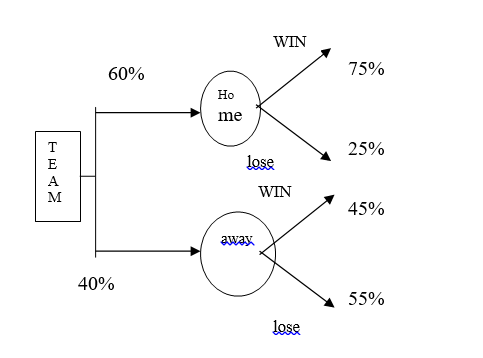A team Plays in Home ground and also outside. 60% plays on Home ground and 40\$ played on Outside. When it played at home ground 75% of matches are won. And 25% are lost. When Game was played by team outside 45% of matches are Won and 55% are lost.

Now Team ins WON this is Given.

Probability in more win are at home ground

P(H/W)=?

P(H/W)=P(W/H)*P(H)

------------------

P(W/H)*P(H)+P(W/H)’*P(H)’

P(W/H)è probability of winning in home series

P(H)    è  probability of games played in Home series

P(W/H)’è probability of winning in outside

P(H)’    è  probability of games played outside

Substitute the values

0.75*0.6

---------------------------

(0.75*0.6)+(0.45*0.4)

=      0.45                        0.45

--------------------  = ------------

0.45+0.18                0.63

= 71.4% probability if our team is won then played in home ground

##### Share on other sites
• 0

Prior probability doesn't consider all background information for predicting an event to happen. Posterior probability considers all background information to calculate probability of event.

An example of Bayes in Retail e-commerce is based on user profile and searches predict the product he/she is looking for and make recommendations.

##### Share on other sites
• 0

Bayes theorem is highly applicable in business scenarios wherever we want to find the probability of occurrence of any event when we have certain clues and guides regarding the processes impacting the outcome of happening of any event. Bayes theorem is closely associated with the Prior and Posterior probability in which the all the evidence and data associated with the occurrence of an event is well known in advance and that is primarily used to calculate the probability of occurrence of an event.

One of the example associated with the manufacturing of textile machinery wherein the Bayes theorem applicability can be tested is: the consumption or procurement of the textile machinery are dependent on several factors. Lets say the most important factors among all those is the tax exemption announced by the Ministry of textiles for textile promotion. This is one of the probability with which the Original Equipment manufacturer can determine the probability of selling of the textile machinery.

Thus Bayes theorem is associated with the degree of belief of a certain process to achieve certain specification. It can be accounted in two scenarios : Pre and Post gathering the evidence.  once the probability is calculated before gathering the evidence it is called is Prior probability calculation and in case, probability is calculated after gathering the evidence, it is called posterior probability calculation.

##### Share on other sites
• 0

The chosen best answer is that of Sharad Talvalkar for providing clear explanation and multiple examples. For more examples, refer to Manjula and Asef's answers and for simple understanding of the concept, read through Sreyash and Ram's answers.

##### Share on other sitesThis topic is now closed to further replies.
• ### Who's Online (See full list)

There are no registered users currently online

• ### Forum Statistics

• Total Topics
2,896
• Total Posts
14,657
• ### Member Statistics

• Total Members
55,433
• Most Online
888

×
×
• Create New...